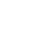Search

# Calculator - Effective Interest Rate

 Effective Rate of Interest Calculated in Accordance with Schedule 2 to the Money Lenders Ordinance (Cap. 163)Particulars of Loans (HK\$) Amount Borrowed (A) 111 Amount Deducted (Interest, Services charges, etc.) (B) 222 Amount of Principal (C )=(A)-(B) -- Amount payable per instalment (D) 333 No. of instalment (E) 444 Interval of each instalment (i.e. Interval between successive payments) (1a) Units 555 Month Week (1b) Is the interval in complete month(s)? 666 Yes No (2a) Number of month(s) 777 (2b) Number of days of incomplete month(s) 888 i.e. -- month(s) Total Amount Payable (F) = (D) x (E) -- Total Amount of Interest (G) = (F) - (C) -- Effective Rate of Interest Calculated in Accordance with Paragraph 4 of Schedule 2 --- per annum